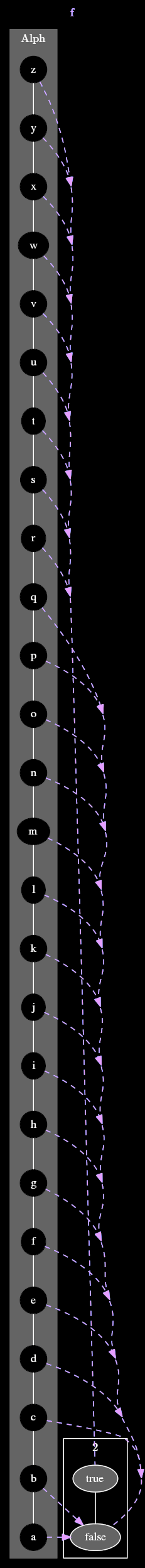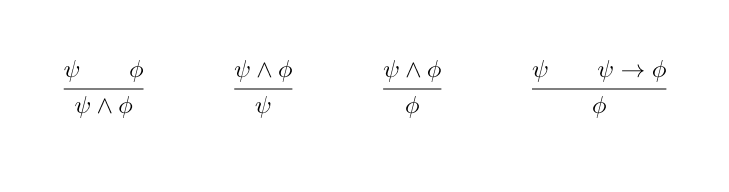# Monotone function: examples

Here are some examples of monotone functions.

## A cunning plan

There’s a two-player game called 10 questions, in which player A begins the game by stating “I’m thinking of an X”, where X can be replaced with any noun of player A’s choosing.

Then player B asks player A a series of questions, which player A must answer with either truthfully with “yes” or “no”. After asking 10 questions, player B is forced to guess what the object player A was thinking of. Player B wins if the guess is correct, and loses otherwise. Player B may guess before 10 turns have expired, in which case the guess counts as one of their questions.

Here is an example of a game of 10 questions:

A: I’m thinking of a food.

Question 1. B: Is it healthy? A: Yes.

Question 2. B: Is it crunchy? A: No.

Question 3. B: Must it be prepared before it is eaten? A: No.

Question 4. B: Is yellow? A: Yes.

Question 5. B: Is it a banana? A: Yes.

Player B wins

Now suppose that you are playing 10 questions as player B. Player A begins by stating “I’m thinking of a letter of the alphabet.” You immediately think of a strategy that requires no more than 5 guesses: repeatedly ask “does it come after $$\star$$?” where $$\star$$ is near the middle of the contiguous sequence of letters that you have not yet eliminated. Initially the contiguous sequence of letters between A and Z have not been eliminated.

Question 1. You: Does it come after M? Player A: Yes.

At this point, the contiguous sequence of 13 letters that have not been eliminated is N-Z, inclusive.

Question 2. You: Does it come after S? Player A: No.

At this point, the contiguous sequence of 6 letters that have not been eliminated is N-S, inclusive.

Question 3. You: Does it come after P? Player A: Yes.

We’ve now narrowed it down to Q,R,and S.

Question 4. You: Does it come after R? Player A: No.

Question 5. You: Does it come after Q? Player A: No.

You: Is it Q? Player A: Yes.

You win

But what does this have to do with monotone functions? The letters of the alphabet form a poset $$Alph = \langle \{A,...,Z\}, \leq_{Alph} \rangle$$ (in fact, a totally ordered set) under the standard alphabetic order. Your strategy for playing 10 questions can be viewed as probing a monotone function from $$Alph$$ to the 2-element poset 2 of a boolean values, where $$false <{\textbf{2}} true$$. Specifically, the probed function $$f : Alph \to \textbf{2}$$ is defined such that $$f(\star) \doteq Q >_{Alph} \star$$

The monotonicity of $$f$$ is crucial to being able to eliminate possibilities at each step. If we probe $$f$$ at a letter $$\star_1$$ and observe a false result, then any letter $$\star_2$$ less than $$\star_1$$ must map to false as well: $$\star_2 \leq_{Alph} \star_1$$ implies $$f(\star_2) \leq_{\textbf{2}} f(\star_1)$$. Yes, we can demonstrate the aformentioned monotone function with a diagram, but since its size is unwieldy, it has been placed in the following hidden text block.## Deduction systems

deduction systems allow one to infer new judgments from a set of known judgments. They are often specified as a set of rules, in which each rule is represented as a horizontal bar with one or more premises appearing above the bar and a conclusion that can be deduced from those premises appearing below the bar.The above rules form a fragment of propositional logic.$$\phi$$ and $$\psi$$ are used to denote logical statements, called propositions.$$\wedge$$ and $$\to$$ are binary logical operators, each of which maps a pair of propositions to a single proposition.$$\wedge$$ is the and operator: if $$\phi$$ and $$\psi$$ are logical statements, then $$\phi \wedge \psi$$ is the simultaneous assertion of both $$\phi$$ and $$\psi$$. $$\to$$ is the implication operator: $$\phi \to \psi$$ asserts that if we know $$\phi$$ to be true, then we can conclude $$\psi$$ is true as well.

The leftmost rule has two premises $$\phi$$ and $$\psi$$, and concludes from these premises the proposition $$\phi \wedge \psi$$. Invoking a rule to deduce its conclusion from its premises is called applying the rule. A tree of rule applications is called a proof.

Deduction systems are often viewed as proof languages. However, it can also be fruitful to view a deduction system as a function which, given a set of input propositions, produces the set of all propositions that can be concluded from exactly one rule application, using the input propositions as premises. More concretely, letting $$X$$ be the set of propositions, the above set of deduction rules corresponds to the following function $$F : \mathcal P(X) \to \mathcal P(X)$$.

$$F(A) = \\ ~~ \{ \phi \wedge \psi\mid\phi \in A, \psi \in A \} \cup \\ ~~ \{ \phi \mid \phi \wedge \psi \in A \} \cup \\ ~~ \{ \psi \mid \phi \wedge \psi \in A \} \cup \\ ~~ \{ \phi \mid \psi \in A, \psi \to \phi \in A \}$$

$$F$$ is a montone function from the poset $$\langle \mathcal P(X), \subseteq \rangle$$ to itself. The reason that $$F$$ is monotone is that larger input sets give us more ways to apply the rules of our system, yielding larger outputs.

In addition to standard logical notions, deduction systems can be used to describe type systems, program logics, and operational semantics.

A monotone function between two totally ordered sets is called increasing. For example, the graph of a monotone function from the poset $$\langle \mathbb R, \le \rangle$$ to itself has an upward slope. Such functions can be searched efficiently using the binary search algorithm.